# Everything You Need To Know About: COUNT Formula In Excel

May 02, 2023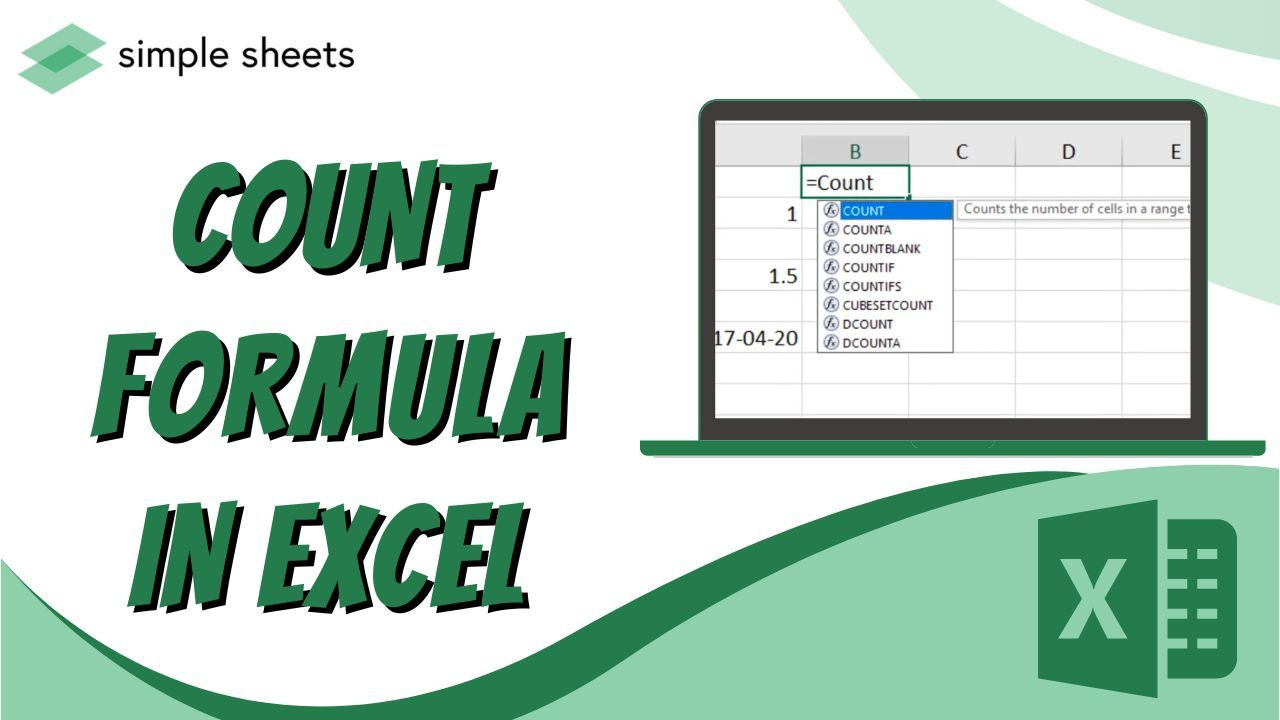Are you ready to discover the advantages of using the COUNT formula in Excel?

If so, you've come to the right place! In this blog post, we will be talking about how to use the COUNT formula in Excel, which can save you time аnd make it much easier for you tо analyze dаtа. So, whether уour goal is to find total occurrences or calculate averages, this formula is the way to go.

Read on as we cover the following:

• The Excel COUNT function

• Excel COUNT Function Important Notes

• Other Excel Features Related to the COUNT Function

• Final Thoughts on COUNT Formula in Excel

• Frequently Asked Questions on COUNT Formula in Excel## The Excel COUNT Function

You use the Excel COUNT function to count cells containing numerical values.

Here's the COUNT function Synxtax: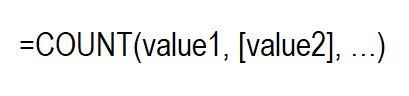To count cells with numbers, specify cell references or ranges such as value1, value2, etc.

The COUNT function in Excel 365-2007 allows a maximum of 255 arguments, while earlier versions of Excel only allow up to 30 values.

Here's an example of how to use a formula to find the total number of numeric cells in the range A2:A101: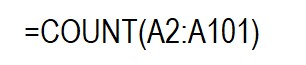Please be aware that the Excel COUNT function counts dates and times stored as serial numbers in the internal Excel system.

## Excel COUNT Function Important Notes

The Excel COUNT function operates according to two simple rules.

1. The Excel Count formula only counts numbers, dates, and times if included as references or in a range. It ignores blank cells and those that contain non-numeric values.

2. Excel's COUNT function can count various types of data, including numbers, dates, times, Boolean values (TRUE and FALSE), and text which includes numbers within quotation marks (i.e., "5"). Enter these values as arguments for the COUNT function in Excel.

The COUNT formula returns a value of 4 after counting the following values: 1, "2", 6/1/2023, and TRUE.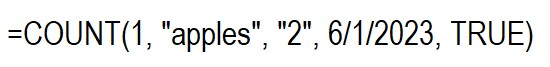### COUNT Function Example

Here are a few more examples of how to use the COUNT function in Excel with various values. To count the numeric cells within a specific range, use this basic COUNT formula: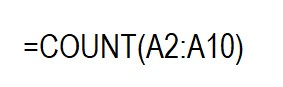The example clearly distinguishes between the types of data that include and those that are out.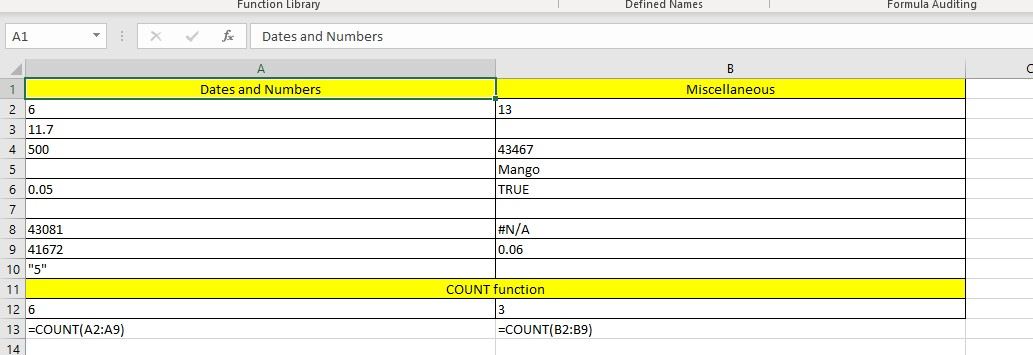To count multiple non-contiguous ranges in Excel, include all ranges in the COUNT formula. For instance, if you want to count cells containing numbers in both columns B and D, you can use a formula like this: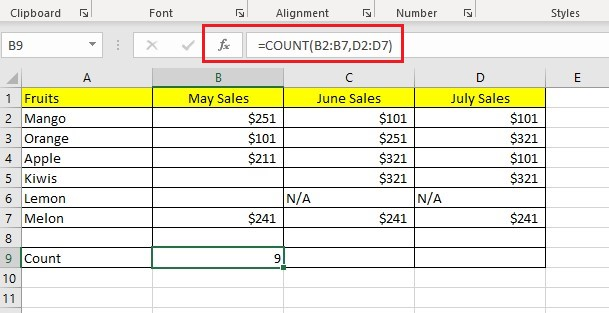Tips:

• To count numbers that meet specific criteria, you can use either the COUNTIF or COUNTIFS function.

• To count logical values, text, errors, and numbers, use the COUNTA function.

## Other Excel Features Related to the COUNT Function

You might need additional COUNT functions in Excel to obtain the correct value in specific scenarios where the regular COUNT function does not suffice.

### COUNTA Function in Excel

The COUNTA function in Excel counts cells that are not empty and contain any value.

The syntax of the Excel COUNTA function is similar to that of the COUNT function.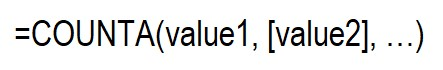To count non-blank cells, use cell references or ranges. Replace 'value1', 'value2', etc., with the appropriate cell references or ranges.

To count the cells that have values in the range A2:A102, use this formula: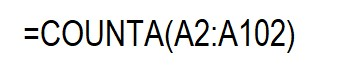To calculate the number of cells that are not empty in multiple ranges that are not next to each other, you can use a COUNTA formula, such as this one: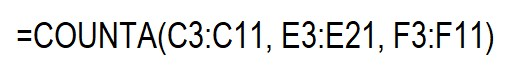You don't need identical sizes for the ranges used in an Excel COUNTA formula, meaning the ranges can have different numbers of rows and columns.

Note that when using Excel's COUNTA function, all types of data are counted, including numbers, dates/times, text values, boolean values (e.g., TRUE and FALSE), and error values (e.g., #VALUE or #N/A), as well as empty text strings (").

Sometimes, the result of the COUNTA function may need to be clarified as it may not match what you see. Even though some cells may appear empty, an Excel COUNTA formula may still count them because they have hidden content.

If you type a space in a cell by mistake or if a cell contains a formula that returns an empty string, both will count.

The only cells not included in the COUNTA function are empty. No other cells are excluded from the count.

Here's a useful tip for counting non-blank cells in Excel:

To count non-empty cells, check the Status Bar at the bottom-right corner of the Excel window; no need for complex formulas or functions. Select the range and look at the Status Bar.

### COUNTIF Function in Excel

The COUNTIF function counts cells that fulfill a specific condition. It needs two arguments that are easy to understand: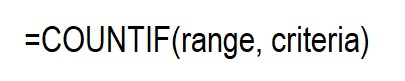In the first parameter, specify the range of cells you wish to count. In the second parameter, indicate the condition you want to use.

To enumerate the number of cells that contain "\$321" in the range B2:D7, you can use the COUNTIF formula below: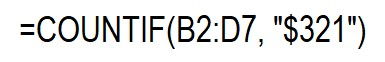To input a criterion, use a cell reference shown in the screenshot. For example, replace the criterion with the cell reference in the formula.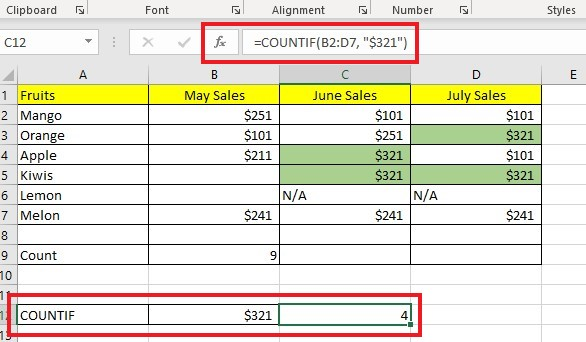### COUNTIFS Function in Excel

To count values based on multiple criteria and ranges, you can use the COUNTIFS function. It has a similar purpose to the COUNTIF function. The syntax for the COUNTIFS function is as follows: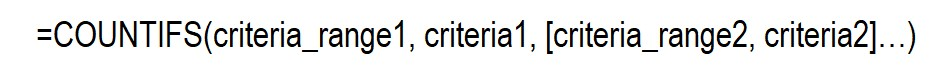The COUNTIFS function is available in Excel 2007 and all later versions, including Excel 2010 through 365.

## Final Thoughts on COUNT Formula in Excel

Using the COUNT formula in Excel is beneficial for data entry and general analytics and provides an incredibly useful way to understand better the information you are analyzing. As seen, it can use to quickly determine how many numbers are there within a range of cells, among other uses.

Visit Simple Sheets for more easy-to-follow guides, and remember to visit the related articles section of this blog post. For the most straightforward Excel video tutorials, subscribe to Simple Sheets on Youtube!## Frequently Asked Questions on COUNT Formula in Excel

### How can I use the COUNTBLANK function in my spreadsheet?

You can use the COUNTBLANK function to count all the blank cells in a range of cells; this is useful if you still need to type data into a cell range.

### How can I count the cells in the selected cell range?

To enumerate the number of cells in a selected range, use the Columns and Rows function. This function counts both the number of columns and rows in an array.

### Why can't I use the COUNTIF function in my spreadsheets?

The error in the COUNTIF function output could be due to a False, duplicate value, or a string with more than 255 characters.## Related Articles:

Excel Countif Function: Simple Guide For Beginners

Basic Microsoft Excel Formulas You NEED to Know

How To Add In Excel in 4 Easy Ways

#### Want to Make Excel Work for You? Try out 5 Amazing Excel Templates & 5 Unique Lessons

We hate SPAM. We will never sell your information, for any reason.